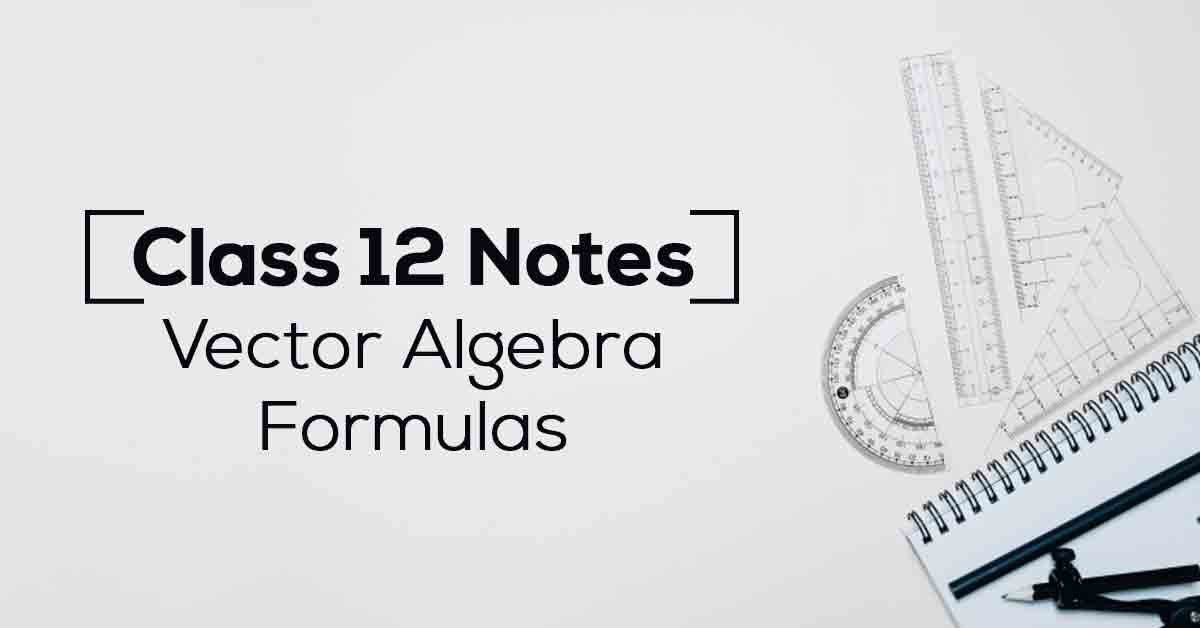Class 12th Math Vector Algebra Formulas CBSE 2023

# Vector Algebra Class 12 Formulas PDF with NotesA vector has magnitude (size) and direction: vector magnitude and direction. The length of the line shows its magnitude and the arrowhead points in the direction. We can add two vectors by joining them head-to-tail: vector add a+b. Know More about these in Vector Algebra Class 12 Formulas PDF with Notes List.

The topics and sub-topics covered in Vector Algebra Class 12 Formulas PDF with Notes are:

10.1 Introduction

10.2 Some Basic Concepts

10.3 Types of Vectors

10.5 Multiplication of a Vector by a Scalar

10.5.1 Components of a vector

10.5.2 Vector joining two points

10.5.3 Section formula

10.6 Product of Two Vectors

10.6.1 Scalar (or dot) product of two vectors

10.6.2 Projection of a vector on a line

10.6.3 Vector (or cross) product of two vectors.

Download the FREE PDF of Vector Algebra Class 12 Formulas PDF with Notes and start your preparation with Vidyakul!